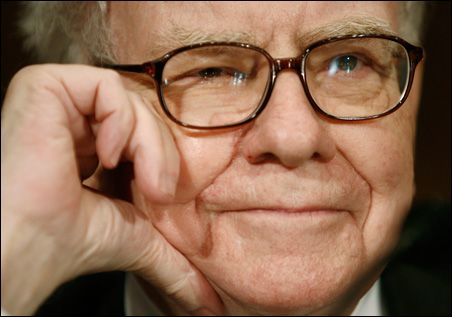Select Page

### Warren Buffet’s Top Positions in Detail

Posted by Guru FocusWarren Buffett – Berkshire Hathaway First Quarter 2012 Fund Portfolio

Here is a current portfolio update of Warren Buffett ‘s Berkshire Hathaway (BRK.A)(BRK.B) portfolio movements as of the end if the first quarter 2012 (March 31, 2012). In total, he has 35 stocks with a total portfolio worth of USD75,300,250,000. Buffett bought two new companies and added seven additional stocks. The biggest influence had Wal-Mart ( WMT ) and Wells Fargo ( WFC ). Both had an impact of more than 0.5 percent of his portfolio. He decreased seven stocks and closed one.

Here are his top positions in detail:

Wells Fargo & Company ( WFC ) has a market capitalization of \$164.41 billion. The company employs 264,900 people, generates revenues of \$49,412.00 million and has a net income of \$16,211.00 million. The firm’s earnings before interest, taxes, depreciation and amortization (EBITDA) amounts to \$31,310.00 million. Because of these figures, the EBITDA margin is 63.37 percent (operating margin 29.22 percent and the net profit margin finally 20.03 percent).

Financial Analysis: The total debt representing 13.28 percent of the company’s assets and the total debt in relation to the equity amounts to 124.39 percent. Due to the financial situation, a return on equity of 12.19 percent was realized. Twelve trailing months earnings per share reached a value of \$2.91. Last fiscal year, the company paid \$0.48 in form of dividends to shareholders.

Market Valuation: Here are the price ratios of the company: The P/E ratio is 10.65, P/S ratio 2.03 and P/B ratio 1.26. Dividend Yield: 2.84 percent. The beta ratio is 1.34.

The Coca-Cola Company ( KO ) has a market capitalization of \$167.20 billion. The company employs 146,200 people, generates revenues of \$46,542.00 million and has a net income of \$8,634.00 million. The firm’s earnings before interest, taxes, depreciation and amortization (EBITDA) amounts to \$12,596.00 million. Because of these figures, the EBITDA margin is 27.06 percent (operating margin 23.06 percent and the net profit margin finally 18.55 percent).

Financial Analysis: The total debt representing 35.72 percent of the company’s assets and the total debt in relation to the equity amounts to 90.31 percent. Due to the financial situation, a return on equity of 27.37 percent was realized. Twelve trailing months earnings per share reached a value of \$3.77. Last fiscal year, the company paid \$1.88 in form of dividends to shareholders.

Market Valuation: Here are the price ratios of the company: The P/E ratio is 19.67, P/S ratio 3.59 and P/B ratio 5.30. Dividend Yield: 2.75 percent. The beta ratio is 0.52.

Intl. Business Machines ( IBM ) has a market capitalization of \$225.94 billion. The company employs 433,362 people, generates revenues of \$106,916.00 million and has a net income of \$15,855.00 million. The firm’s earnings before interest, taxes, depreciation and amortization (EBITDA) amounts to \$26,266.00 million. Because of these figures, the EBITDA margin is 24.57 percent (operating margin 19.64 percent and the net profit margin finally 14.83 percent).

Financial Analysis: The total debt representing 26.90 percent of the company’s assets and the total debt in relation to the equity amounts to 155.54 percent. Due to the financial situation, a return on equity of 73.43 percent was realized. Twelve trailing months earnings per share reached a value of \$13.41. Last fiscal year, the company paid \$2.90 in form of dividends to shareholders.

Market Valuation: Here are the price ratios of the company: The P/E ratio is 14.60, P/S ratio 2.11 and P/B ratio 11.31. Dividend Yield: 1.74 percent. The beta ratio is 0.66.

American Express ( AXP ) has a market capitalization of \$63.76 billion. The company employs 63,700 people, generates revenues of \$32,282.00 million and has a net income of \$4,899.00 million. The firm’s earnings before interest, taxes, depreciation and amortization (EBITDA) amounts to \$10,194.00 million. Because of these figures, the EBITDA margin is 31.58 percent (operating margin 21.55 percent and the net profit margin finally 15.18 percent).

Financial Analysis: The total debt representing 41.08 percent of the company’s assets and the total debt in relation to the equity amounts to 335.18 percent. Due to the financial situation, a return on equity of 27.64 percent was realized. Twelve trailing months earnings per share reached a value of \$4.18. Last fiscal year, the company paid \$0.72 in form of dividends to shareholders.

Market Valuation: Here are the price ratios of the company: The P/E ratio is 13.25, P/S ratio 1.98 and P/B ratio 3.43. Dividend Yield: 1.44 percent. The beta ratio is 1.82.

For an entire list of the holdings in Warren Buffet’s full portfolio go HERE